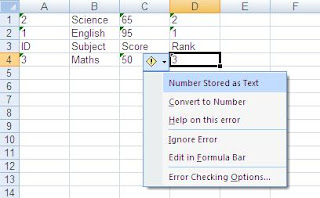# Create New XLS File POI Java Code

We had a very long introduction on reading excel documents using Apache POI in our earlier tutorial. In this example, we will describe how to create / write an XLS document using POI library. We will create the document in Office 97 - 2003 format. We will also discuss a few exceptions that you can possibly get when writing an excel document in this format with POI.

## Create New XLS File - POI Java Code

The complete program to create an XLS file using Apache POI library is provided below. The code is commented so that you can understand this completely.

```import java.io.FileOutputStream;
import java.io.*;
import org.apache.poi.hssf.usermodel.HSSFWorkbook;
import org.apache.poi.hssf.usermodel.HSSFSheet;
import org.apache.poi.ss.usermodel.*;
import java.util.*;
public class createxls {
public static void main(String[] args) throws Exception{
HSSFWorkbook new_workbook = new HSSFWorkbook(); //create a blank workbook object
HSSFSheet sheet = new_workbook.createSheet("Score_Details");  //create a worksheet with caption score_details
Map<String, Object[]> excel_data = new HashMap<String, Object[]>(); //create a map and define data
excel_data.put("1", new Object[] {"ID", "Subject", "Score","Rank"}); //add data
excel_data.put("2", new Object[] {"1", "English", "95","1"});
excel_data.put("3", new Object[] {"2", "Science", "65","2"});
excel_data.put("4", new Object[] {"3", "Maths", "50","3"});
Set<String> keyset = excel_data.keySet();
int rownum = 0;
for (String key : keyset) { //loop through the data and add them to the cell
Row row = sheet.createRow(rownum++);
Object [] objArr = excel_data.get(key);
int cellnum = 0;
for (Object obj : objArr) {
Cell cell = row.createCell(cellnum++);
cell.setCellValue((String)obj);
}
}

FileOutputStream output_file = new FileOutputStream(new File("C:\\POI_XLS_File.xls")); //create XLS file
new_workbook.write(output_file);//write excel document to output stream
output_file.close(); //close the file
}
}
```

This code creates a new Excel document under C:\ drive, the output of which is shown below:

## Create XLS in POI - Sample Output

The output produced by the Java code is shown below:XLS Created in POI - Example Output

Handle "Number Stored as Text"- Warning in POI

If you see the output above, you can find that Excel throws a warning "Number Stored as Text" message when you open the document in Excel after creation. This is not an error, but you can easily get around this problem, by modifying your code to create the spreadsheet as per below: (only an example is provided below)

```                excel_data.put("1", new Object[] {"ID", "Subject", "Score","Rank"}); //add data
excel_data.put("2", new Object[] {"1", "English", "95",1d});
excel_data.put("3", new Object[] {"2", "Science", "65",2d});
excel_data.put("4", new Object[] {"3", "Maths", "50",3d});
Set<String> keyset = excel_data.keySet();
int rownum = 0;
for (String key : keyset) { //loop through the data and add them to the cell
Row row = sheet.createRow(rownum++);
Object [] objArr = excel_data.get(key);
int cellnum = 0;
for (Object obj : objArr) {
Cell cell = row.createCell(cellnum++);
if(obj instanceof Double)
cell.setCellValue((Double)obj);
```Number Stored as Text - Solution in Apache POI
You can handle formats like Date, Boolean on the same lines as above. The properties of the Excel file created in POI using the example above is shown below:Excel File Properties Created in POI
Note: Timestamps are removed.

That completes the tutorial to create XLS document using Apache POI. In the next example, we will discuss how to create XLSX document in Apache POI with relevant examples. Meanwhile, if you have a question on this tutorial, post it in the comments section.

This Tutorial: How to create new XLS document in Java using Apache POI library?
Keywords: Java, Excel 2000 format, Apache POI, ,Create XLS in Java, Example Program
Next Tutorial: How to create XLSX files in Java using Apache POI.